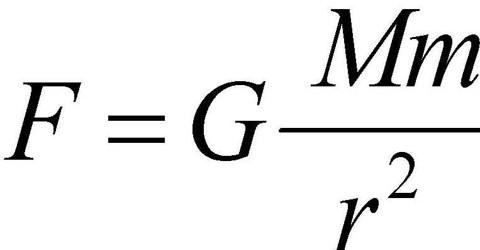# Gravitational Constant

Gravitational Constant

According to Newton’s law of gravitation, force of attraction between two bodies having masses M and m is,

F = GMm/d2, here G = gravitational constant and d = distance between the two bodies.

Suppose, mass of each body is unity and the distance between them is also unity i.e., M =1 unit, m = 1 unit and d = 1 unit then,

F = (G x 1 x 1) / (1 x 1)

or, F = G … … … (1)

From equation (1) gravitational constant may be defined in the following way:

Gravitational constant may be defined to be numerically equal to the force of attraction between two unit masses kept at unit distance apart. The value of G does not depend on mass of the body nor on the distance from the centre of mass to the distance of the body.

In S. I. unit its value is 6.67 x 10-11 N-m2/kg-2.

Dimension of gravitational constant: (G) = (M-1T-2L-3)

Gravitational constant, G is called universal constant.